# ISEE Middle Level Math : How to find the decimal equivalent of a fraction

## Example Questions

### Example Question #21 : Fractions

Which is the decimal equivalent of 45%?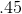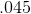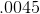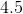Explanation:

Find the equivalent by dividing: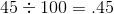### Example Question #21 : How To Find The Decimal Equivalent Of A Fraction

Find the decimal equivalent of 95%.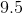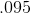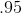Explanation:

Divide: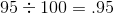Answer:### Example Question #22 : How To Find The Decimal Equivalent Of A Fraction

Find the decimal equivalent of 33%.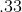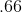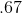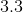Explanation:

Divide: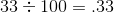### Example Question #23 : How To Find The Decimal Equivalent Of A Fraction

Find the decimal equivalent of 58%.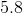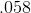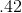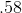Explanation:

Divide: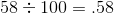### Example Question #24 : How To Find The Decimal Equivalent Of A Fraction

Which of these is equivalent to 29%?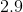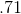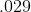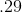Explanation:

Divide: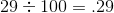Answer:### Example Question #25 : How To Find The Decimal Equivalent Of A Fraction

Which of these is equivalent to 83%?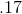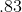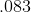Explanation:

Divide: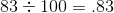Answer:### Example Question #22 : Fractions

Express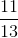as a decimal.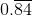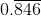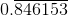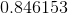Explanation:

Divide 11 by 13: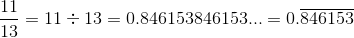### Example Question #28 : Fractions

Express as a decimal: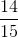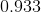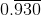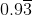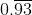Explanation:

Divide 14 by 15: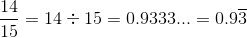### Example Question #174 : Numbers And Operations

Express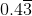as a fraction in lowest terms.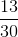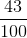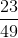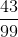Explanation:

Set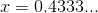Then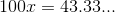and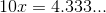Subtract: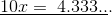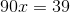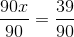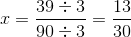### Example Question #24 : How To Find The Decimal Equivalent Of A Fraction

Express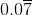as a fraction in lowest terms.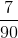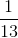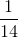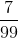Explanation:

Let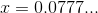Then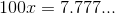and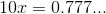Subtract: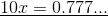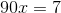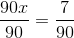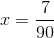### All ISEE Middle Level Math Resources